# Common Core: High School - Geometry : Construct Inscribed Figures (Equilateral Triangles, Squares, Regular Hexagons)

## Example Questions

← Previous 1 3

### Example Question #1 : Construct Inscribed Figures (Equilateral Triangles, Squares, Regular Hexagons): Ccss.Math.Content.Hsg Co.D.13

Given only the line segment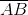, what is the first step to construct a diamond.

Draw a parallel line from pointof length.

Calculate the length of.

Draw a perpendicular line from pointof length.

Draw aangle.

Calculate the slope of.

Calculate the length of.

Explanation:

Given only a line segment there are numerous steps that need to be taken to construct a diamond. Recall a diamond is a parallelogram with four equilateral sides where the diagonals are vertical and horizontal.

The first step in constructing a diamond would be to calculate the length of the line segment. After the length is known then the slope can be found and from there, each of the connecting lines can be drawn.

"Calculate the length of."

### Example Question #1 : Construct Inscribed Figures (Equilateral Triangles, Squares, Regular Hexagons): Ccss.Math.Content.Hsg Co.D.13

Which of the following images is a triangle that is inscribed in a circle?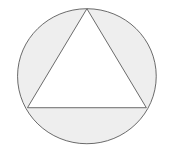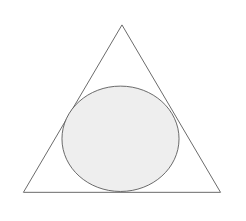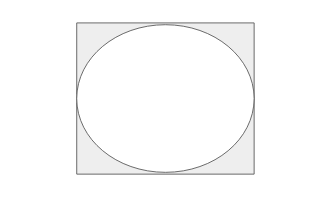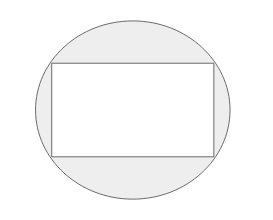Explanation:

To determine which image illustrates a triangle that is inscribed in a circle, first understand what the term "inscribed" means.

"Inscribed" means to draw inside of. Therefore, a triangle inscribed in a circle means the triangle will be drawn inside of a circle.

The image that illustrates this is as followed.### Example Question #2 : Construct Inscribed Figures (Equilateral Triangles, Squares, Regular Hexagons): Ccss.Math.Content.Hsg Co.D.13

Which of the following images is a rectangle that is inscribed in a circle?Explanation:

To determine which image illustrates a rectangle that is inscribed in a circle, first understand what the term "inscribed" means.

"Inscribed" means to draw inside of. Therefore, a rectangle inscribed in a circle means the rectangle will be drawn inside of a circle.

The image that illustrates this is as followed.### Example Question #3 : Construct Inscribed Figures (Equilateral Triangles, Squares, Regular Hexagons): Ccss.Math.Content.Hsg Co.D.13

Which of the following images is a circle that is inscribed in a rectangle?Explanation:

To determine which image illustrates a circle that is inscribed in a rectangle, first understand what the term "inscribed" means.

"Inscribed" means to draw inside of. Therefore, a circle inscribed in a rectangle means the circle will be drawn inside of a rectangle.

The image that illustrates this is as followed.### Example Question #4 : Construct Inscribed Figures (Equilateral Triangles, Squares, Regular Hexagons): Ccss.Math.Content.Hsg Co.D.13

Which of the following images is a circle that is inscribed in a triangle?Explanation:

To determine which image illustrates a circle that is inscribed in a triangle, first understand what the term "inscribed" means.

"Inscribed" means to draw inside of. Therefore, a circle inscribed in a triangle means the circle will be drawn inside of a triangle.

The image that illustrates this is as followed.### Example Question #5 : Construct Inscribed Figures (Equilateral Triangles, Squares, Regular Hexagons): Ccss.Math.Content.Hsg Co.D.13

Which of the following images is a pentagon that is inscribed in a circle?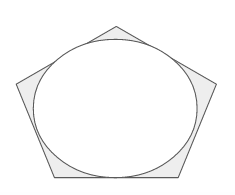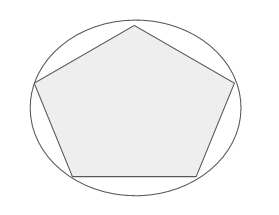Explanation:

To determine which image illustrates a pentagon that is inscribed in a circle, first understand what the term "inscribed" means.

"Inscribed" means to draw inside of. Therefore, a pentagon inscribed in a circle means the pentagon will be drawn inside of a circle.

The image that illustrates this is as followed.### Example Question #6 : Construct Inscribed Figures (Equilateral Triangles, Squares, Regular Hexagons): Ccss.Math.Content.Hsg Co.D.13

Which of the following images is a circle that is inscribed in a pentagon?Explanation:

To determine which image illustrates a circle that is inscribed in a pentagon, first understand what the term "inscribed" means.

"Inscribed" means to draw inside of. Therefore, a circle inscribed in a pentagon means the circle will be drawn inside of a pentagon.

The image that illustrates this is as followed.### Example Question #140 : Congruence

If a rectangle is inscribed in a circle what can be said about the relationship between the circles diameter and a measurement on the rectangle?

The diameter of the circle is equal to the diagonal of the rectangle.

The diameter of the circle is equal to the perimeter of the rectangle.

All of the answers are equivalencies.

The diameter of the circle is equal to the side length of the rectangle.

The diameter of the circle is equal to the side squared of the rectangle.

The diameter of the circle is equal to the diagonal of the rectangle.

Explanation:

First understand what the term "inscribed" means.

"Inscribed" means to draw inside of. Therefore, a rectangle inscribed in a circle means the rectangle will be drawn inside of a circle.

From here the following image can be constructed.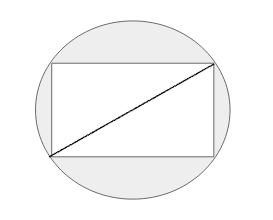The diameter of the circle is any and all straight lines that cut the circle in half. Since the rectangle is inscribed in that circle and all corners touch the circle then the diameter of the circle is equivalent to the length of the rectangle's diagonal.

### Example Question #2 : Construct Inscribed Figures (Equilateral Triangles, Squares, Regular Hexagons): Ccss.Math.Content.Hsg Co.D.13

Determine whether the statement is true or false:

For an object to be inscribed in a circle only one of its points must lie within the circle.

False

True

False

Explanation:

To determine whether this particular statement is true or false, first understand what the term "inscribed" means.

"Inscribed" means to draw inside of. Therefore, for any object to be inscribed in a circle that means all points of that object must also be inside the circle.

Thus the statement, "For an object to be inscribed in a circle only one of its points must lie within the circle." is false.

### Example Question #3 : Construct Inscribed Figures (Equilateral Triangles, Squares, Regular Hexagons): Ccss.Math.Content.Hsg Co.D.13

Determine whether the statement is true or false:

Polygons can be both inscribed inside a circle and have circles inscribed inside of them.

False

True

True

Explanation:

To determine whether this particular statement is true or false, first understand what the term "inscribed" means.

"Inscribed" means to draw inside of. Therefore, any and all polygons can be inscribed in a circle and circles can be inscribed in all polygons.

Thus, the statement, "Polygons can be both inscribed inside a circle and have circles inscribed inside of them." is true.

← Previous 1 3

### All Common Core: High School - Geometry Resources